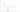## 吃豆子的wayne

Fri, 24th January 2020Edit on Github豆子算法

# 问题提出

2013年2月KeyTo9_Fans提出了wayne吃豆子问题: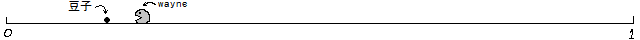wayne的移动速度是每秒$1$单位长度。
wayne可以随时改变移动方向。
wayne总是采取最佳策略，使得吃豆子的平均速率最大。

$k=1$时，wayne吃豆子的平均速率是$3$个每秒。

# 初步展开

wayne认为:

KeyTo9_Fans指出, 问题没有这么简单:
$k=1$当然没问题咯，豆子在哪边wayne就往哪边跑。

wayne经常要思考“到底先吃哪边的豆子才能使得吃豆子的平均速率最大呢？”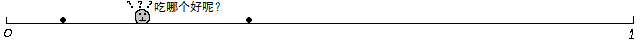wayne吃豆子的平均速率是$(4.53704\pm 0.00002)$个每秒。

“一旦wayne把一个豆子吃掉，一个新的豆子就会立即出现在$[0,1]$区间中均匀分布的一个随机的点上。”
wayne最初的位置在哪里都不要紧。

$\lim_{t\to\infty}\frac{n(t)}t$的值都是一样的.

$k=2$时，wayne的策略可以用一个$3$元的布尔函数$f$来描述。
$f$的输入是$x_1$$x_2$$x_w$

$f$的输出是一个方向，“左”或者“右”。

$\frac{n(t)}t$越大，说明wayne的策略越好，$\frac{n(t)}t$越小，说明wayne的策略越差。

# 最近策略不必然最优

KeyTo9_Fans通过实验的方法发现，当$k=2$时，“总是吃最近的豆子”并不是最佳策略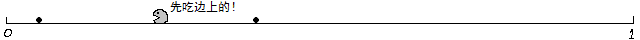$Score(x_i)=\frac{|x_i-\frac12|+\frac12}{|x_w-x_i|}$，（表示豆子离$\frac12$处越远越好，离wayne越近越好）
$Go(x_w\to x_i)$表示$x_i$$x_w$的哪边就去哪边。

$3$楼“总是吃最近的豆子”的策略的速率只有$(4.53704\pm0.00002)$个每秒） wayne建议Score函数里面的参数$\frac12$可以替换成其它值再试验一下看看。
Fans经过试验，得出:

# 不同权函数试验

mathe认为:

zgg__参考mathe的思路，尝试了一些函数g，最后还是选择了平均数，令gq(x1,x2)为x1、x2和$\frac12$这三个数的加权算数平均数，其权的比例为1：1：q，故$gq(x1,x2)=\frac{x1+x2+\frac q2}{q+2}$。然后做了一点数据模拟，对每一个k，每次吃$10^5$个豆，反复吃50次求平均、最大和最小（代码见下）。

g[x1_, x2_, k_] := (x1 + x2 + k/2)/(k + 2);
ks = {};
Do[ts = {};
Do[w = 0.5; t = 0; s1 = Random[]; s2 = Random[];
Do[If[s1 < s2, x1 = s1; x2 = s2, x1 = s2; x2 = s1;];
If[w < g[x1, x2, k], tt = Abs[w - x1]; w = x1; s1 = x2;,
tt = Abs[w - x2]; w = x2; s1 = x1;];
s2 = Random[]; t = (t n + tt)/(n + 1);, {n, 0, 100000}];
AppendTo[ts, t];, {50}];
AppendTo[ks, {{k, Mean[ts]}, {k, Min[ts]}, {k, Max[ts]}}];
Print[k];, {k, 0, 2, 0.1}];
ListPlot[Transpose[ks], Filling -> {1 -> {2}, 1 -> {3}},
PlotMarkers -> {" \[FilledCircle]", "\[LongDash]", "\[LongDash]"}]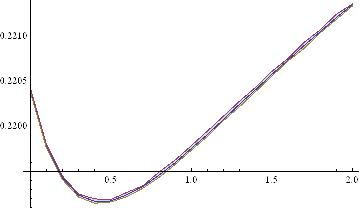dianyancao也给出了他的一种策略:

p=0.38
t=0.218766
v1 = Abs[w - rightx] + p * Abs[leftx - xforecast];
v2 = Abs[w - leftx] + p * Abs[rightx - xforecast];

rightx位于w右边，leftx位于w左边，xforecast=1/2 根据v1和v2的大小关系，确定吃右边的豆子还是左边的豆子 已经对应的Mathematica代码和结果图: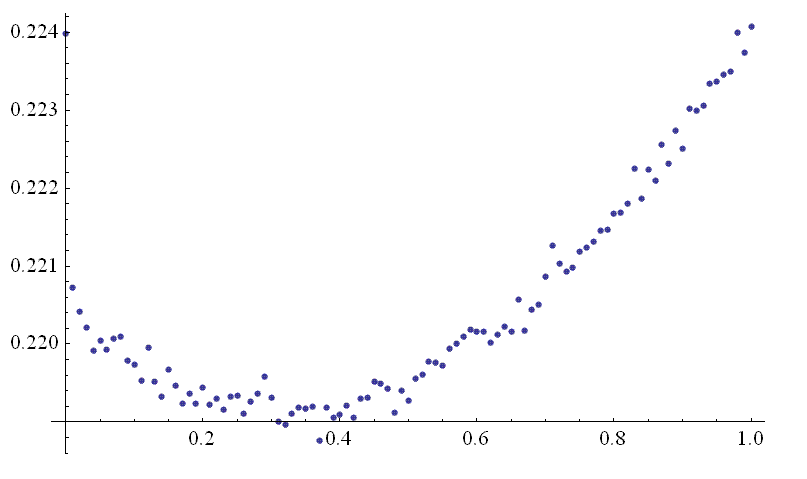# 采用积分计算

mathe提议，k=2时设最优策略是g(a,b),也就是在豆子位置为$x_1\lt x_2$，如果$w,选择$x_1$位置的豆子，不然另外一个豆子。那么设稳定时豆子和wayne位置的密度分布函数为$p(x_1,x_2,w)$
$K(a,b)=\int_0^{g(a,b)} p(a,b,w)dw, F(a,b)=\int_{g(a,b)}^1 p(a,b,w)dw$

zgg继续结合他的权函数，采用积分算式计算: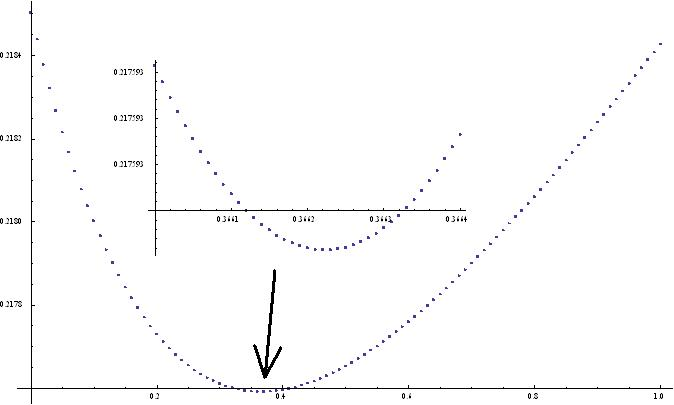mathe对最简单的权函数$g(a,b)=\frac{a+b}2$进行积分运算，分别给出了两个不同版本的Pari/gp代码: 代码1代码2.

(16:06) gp > getdist(2)
%17 = 0.2114583333333333333333333333
(16:07) gp > getdist(10)
%18 = 0.2203686807254583318975768873
(16:07) gp > getdist(20)
%19 = 0.2204074196271448826430741941
(16:07) gp > getdist(30)
%20 = 0.2204077318388700882261725891
(16:07) gp > getdist(40)
%21 = 0.2204077356077135199957228968
(16:07) gp > getdist(50)
%22 = 0.2204077356585942289207798388
(16:07) gp > getdist(60)
%23 = 0.2204077356593274980124179385
(16:07) gp > getdist(70)
%25 = 0.2204077356593385397246878697
(16:33) gp > getdist(99)
%1 = 0.2204077356593387142224517526
(16:10) gp > getdist(100)
%26 = 0.2204077356593387141426361209

# k=2时结果总结

KeyTo9_Fans对多种策略进行模拟比较:

$g(x_1,x_2)=\frac{x_1+x_2}2$ $t=0.220408(1)$
$Score(x)=\frac{\mid x-\frac12\mid+a}{\mid w-x\mid}$ $a=0.523(5),t=0.2194662(5)$
$Score(x)=\mid w-x^{\prime}\mid +p\mid x-\frac12\mid$ $p=0.311(5),t=0.2191733(5)$
$g(x_1,x_2)=\frac{x_1+x_2+\frac p2}{2+p}$ $p=0.478(5),t=0.2191031(5)$
$g(x_1,x_2)=\frac{\max\{x_1,x_2\}}{1+\mid x_1-x_2\mid}$ $t=0.2190061(5)$
$g(x_1,x_2)=\frac{x_1+x_2+\frac p2\mid x_1-x_2\mid}{2+p\mid x_1-x_2\mid }$ $p=1.753(5),t=0.2189915(5)$
$g(x_1,x_2)=\frac{x_1+x_2+\frac p2\mid x_1-x_2\mid ^q}{2+p\mid x_1-x_2\mid ^q}$ $p=1.28(3),q=0.72(2),t=0.2189685(5)$

Fans终于得到了最佳策略下的平均吃豆时长，
$0.21889630(1)$

Fans将该结果列在了上表的最后一行，

$k=2$时，wayne吃豆子的平均速率是$4.5683732(2)$个每秒。

Fans把这个问题做成了一个游戏

collect.exe”放在同一个文件夹里，不要分开了。

Github